# Mixed-Precision Programming with CUDA 8Update, March 25, 2019: The latest Volta and Turing GPUs now incoporate Tensor Cores, which accelerate certain types of FP16 matrix math. This enables faster and easier mixed-precision computation within popular AI frameworks. Making use of Tensor Cores requires using CUDA 9 or later. NVIDIA has also added automatic mixed precision capabilities to TensorFlow,  PyTorch, and MXNet. Interested in learning more or trying it out for yourself? Get tensor core optimized examples for popular AI frameworks here.

In the practice of software development, programmers learn early and often the importance of using the right tool for the job. This is especially important when it comes to numerical computing, where tradeoffs between precision, accuracy, and performance make it essential to choose the best representations for data. With the introduction of the Pascal GPU architecture and CUDA 8, NVIDIA is expanding the set of tools available for mixed-precision computing with new 16-bit floating point and 8/16-bit integer computing capabilities.

“As the relative costs and ease of computing at different precisions evolve, due to changing architectures and software, as well as the disruptive influence of accelerators such as GPUs, we will see an increasing development and use of mixed precision algorithms.” — Nick Higham, Richardson Professor of Applied Mathematics, University of Manchester

Many technical and HPC applications require high precision computation with 32-bit (single float, or FP32) or 64-bit (double float, or FP64) floating point, and there are even GPU-accelerated applications that rely on even higher precision (128- or 256-bit floating point!). But there are many applications for which much lower precision arithmetic suffices. For example, researchers in the rapidly growing field of deep learning have found that deep neural network architectures have a natural resilience to errors due to the backpropagation algorithm used in training them, and some have argued that 16-bit floating point (half precision, or FP16) is sufficient for training neural networks.

Storing FP16 (half precision) data compared to higher precision FP32 or FP64 reduces memory usage of the neural network, allowing training and deployment of larger networks, and FP16 data transfers take less time than FP32 or FP64 transfers. Moreover, for many networks deep learning inference can be performed using 8-bit integer computations without significant impact on accuracy.

In addition to deep learning, applications that use data from cameras or other real-world sensors often don’t require high-precision floating point computation, because the sensors generate low-precision or low dynamic range data. The data processed from radio telescopes is a good example. As you’ll see later in this post, the cross correlation algorithm used for processing data from radio telescopes can be greatly accelerated by using 8-bit integer computation.

The combined use of different numerical precisions in a computational method is known as mixed precision. The NVIDIA Pascal architecture provides features aimed at providing even higher performance for applications that can utilize lower precision computation, by adding vector instructions that pack multiple operations into a 32-bit datapath. Specifically, these instructions operate on 16-bit floating point data (“half” or FP16) and 8- and 16-bit integer data (INT8 and INT16).

The new NVIDIA Tesla P100, powered by the GP100 GPU, can perform FP16 arithmetic at twice the throughput of FP32. The GP102 (Tesla P40 and NVIDIA Titan X), GP104 (Tesla P4), and GP106 GPUs all support instructions that can perform integer dot products on 2- and4-element 8-bit vectors, with accumulation into a 32-bit integer. These instructions are valuable for implementing high-efficiency deep learning inference, as well as other applications such as radio astronomy.

In this post I will provide some details about half-precision floating point, and provide details about the performance achievable on Pascal GPUs using FP16 and INT8 vector computation. I will also discuss the mixed-precision computation capabilities provided by various CUDA platform libraries and APIs.

## A Bit (or 16) about Floating Point Precision

As every computer scientist should know, floating point numbers provide a representation that allows real numbers to be approximated on a computer with a tradeoff between range and precision. Floating point numbers approximate the real value to a set number of significant digits, known as the mantissa or significand, and then scaled by an exponent in a fixed base (base 2 for IEEE standard floating point numbers used on most computers today).

Common floating point formats include 32-bit, known as “single precision” (`float` in C-derived programming languages), and 64-bit, known as “double precision” (`double`). As defined by the IEEE 754 standard, a 32-bit floating point value comprises a sign bit, 8 exponent bits, and 23 mantissa bits. A 64-bit double comprises a sign bit, 11 exponent bits, and 52 mantissa bits. In this post, we’re interested in the (newer) IEEE 754 standard 16-bit floating half type, which comprises a sign bit, 5 exponent bits, and 10 mantissa bits, as Figure 1 shows.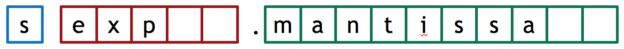Figure 1: 16-bit half-precision floating point (FP16) representation: 1 sign bit, 5 exponent bits, and 10 mantissa bits.

To get an idea of what a difference in precision 16 bits can make, FP16 can represent 1024 values for each power of 2 between 2-14 and 215 (its exponent range). That’s 30,720 values. Contrast this to FP32, which can represent about 8 million values for each power of 2 between 2-126 and 2127. That’s about 2 billion values—a big difference. So why use a small floating-point format like FP16? In a word, performance.

The NVIDIA Tesla P100 (based on the GP100 GPU) supports a 2-way vector half-precision fused multiply-add (FMA) instruction (opcode HFMA2), which it can issue at the same rate as 32-bit FMA instructions. This means that half-precision arithmetic has twice the throughput of single-precision arithmetic on P100, and four times the throughput of double precision. Specifically, the NVLink-enabled P100 (SXM2 module) is capable of 21.2 Teraflop/s of half-precision. With such a big performance benefit, it’s worth looking at how you can use it.

One thing to keep in mind when using reduced precision is that because the normalized range of FP16 is smaller, the probability of generating subnormal numbers (also known as denormals) increases. Therefore it’s important that NVIDIA GPUs implement FMA operations on subnormal numbers with full performance. Some processors do not, and performance can suffer. (Note: you may still see benefits from enabling “flush to zero”. See the post “CUDA Pro Tip: Flush Denormals with Confidence”.)

## High Performance with Low-Precision Integers

Floating point numbers combine high dynamic range with high precision, but there are also cases where dynamic range is not necessary, so that integers may do the job. There are even applications where the data being processed has low precision so very low-precision storage (such as C short or char/byte types) can be used.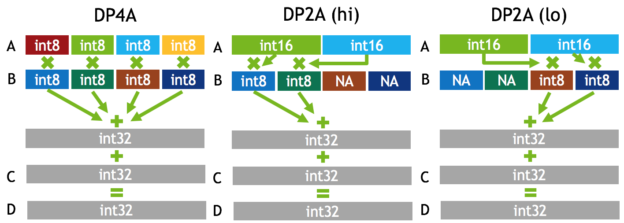Figure 2: New DP4A and DP2A instructions in Tesla P4 and P40 GPUs provide fast 2- and 4-way 8-bit/16-bit integer vector dot products with 32-bit integer accumulation.

For such applications, the latest Pascal GPUs (GP102, GP104, and GP106) introduce new 8-bit integer 4-element vector dot product (DP4A) and 16-bit 2-element vector dot product (DP2A) instructions. DP4A performs the vector dot product between two 4-element vectors A and B (each comprising 4 single-byte values stored in a 32-bit word), storing the result in a 32-bit integer, and adding it to a third argument C, also a 32-bit integer. See Figure 2 for a diagram. DP2A is a similar instruction in which A is a 2-element vector of 16-bit values and B is a 4-element vector of 8-bit values, and different flavors of DP2A select either the high or low pair of bytes for the 2-way dot product. These flexible instructions are useful for linear algebraic computations such as matrix multiplies and convolutions. They are particularly powerful for implementing 8-bit integer convolutions for deep learning inference, common in the deployment of deep neural networks used for image classification and object detection. Figure 3 shows the improved power efficiency achieved on a Tesla P4 GPU using INT8 convolution on AlexNet.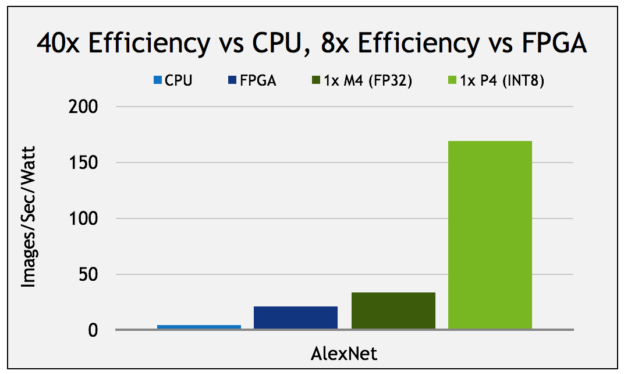Figure 3: Using INT8 computation on the Tesla P4 for deep learning inference provides a very large improvement in power efficiency for image recognition using AlexNet and other deep neural networks, when compared to FP32 on previous generation Tesla M4 GPUs. Efficiency of this computation on Tesla P4 is up to 8x more efficient than an Arria10 FPGA, and up to 40x more efficient than an Intel Xeon CPU. (AlexNet, batch size = 128, CPU: Intel E5-2690v4 using Intel MKL 2017, FPGA is Arria10-115. 1x M4/P4 in node, P4 board power at 56W, P4 GPU power at 36W, M4 board power at 57W, M4 GPU power at 39W, Perf/W chart using GPU power.)

DP4A computes the equivalent of a total of eight integer operations, and DP2A computes four. This gives the Tesla P40 (based on GP102) a peak integer throughput of 47 TOP/s (Tera operations per second).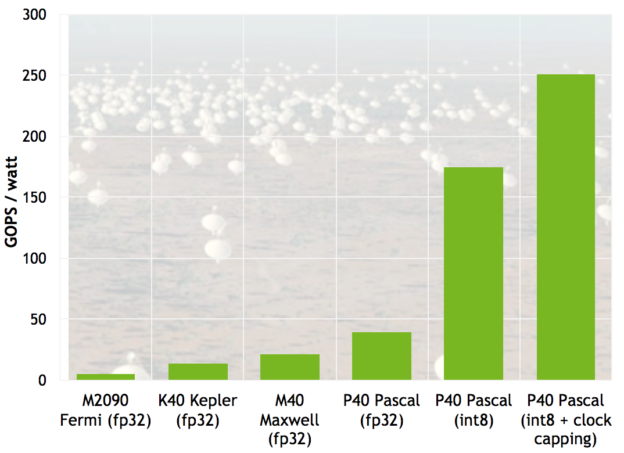Figure 4: INT8 vector dot products (DP4A) improve the efficiency of radio astronomy cross-correlation by a large factor compared to FP32 computation.

## Mixed Precision Performance on Pascal GPUs

The half precision (FP16) Format is not new to GPUs. In fact, FP16 has been supported as a storage format for many years on NVIDIA GPUs, mostly used for reduced precision floating point texture storage and filtering and other special-purpose operations. The Pascal GPU architecture implements general-purpose, IEEE 754 FP16 arithmetic. High performance FP16 is supported at full speed on Tesla P100 (GP100), and at lower throughput (similar to double precision) on other Pascal GPUs (GP102, GP104, and GP106), as the following table shows.

The 8-bit and 16-bit DP4A and DP2A dot product instructions are supported on GP102-GP106, but not on GP100. Table 1 shows the arithmetic throughput of the different numerical instructions on Pascal-based Tesla GPUs.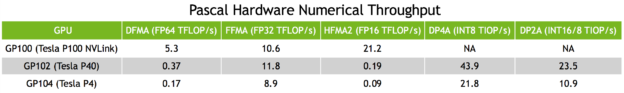Table 1: Pascal-based Tesla GPU peak arithmetic throughput for half-, single-, and double-precision fused multiply-add instructions, and for 8- and 16-bit vector dot product instructions. (Boost clock rates are used in calculating peak throughputs. TFLOP/s: Tera Floating-point Operations per Second. TIOP/s: Tera Integer Operations per Second.

## Mixed-Precision Programming with NVIDIA Libraries

The easiest way to benefit from mixed precision in your application is to take advantage of the support for FP16 and INT8 computation in NVIDIA GPU libraries. Key libraries from the NVIDIA SDK now support a variety of precisions for both computation and storage.

Table 2 shows the current support for FP16 and INT8 in key CUDA libraries as well as in PTX assembly and CUDA C/C++ intrinsics.

### cuDNNcuDNN is a library of primitive routines used in training and deploying deep neural networks. cuDNN 5.0 includes FP16 support for forward convolution, and 5.1 added support for FP16 backward convolution. All other routines in the library are memory bound, so FP16 computation is not beneficial to performance. Therefore these routines use FP32 computation but support FP16 data input and output. cuDNN 6 will add support for INT8 inference convolutions.

### TensorRT

TensorRT is a high-performance deep learning inference engine for production deployment of deep learning applications  that automatically optimizes trained neural networks for run-time performance. TensorRT v1 has support for FP16 for inference convolutions, and v2 will support INT8 for inference convolutions.

### cuBLAS

cuBLAS is a GPU library for dense linear algebra— an implementation of BLAS, the Basic Linear Algebra Subroutines. cuBLAS has support for mixed precision in several matrix-matrix multiplication routines. cublasHgemm is a FP16 dense matrix-matrix multiply routine that uses FP16 for compute as well as for input and output. cublasSgemmEx() computes in FP32, but the input data can be FP32, FP16, or INT8, and the output can be FP32 or FP16. cublasGemm() is a new routine in CUDA 8 that allows specification of the computation precision, including INT8 computation (which uses DP4A).

Support for more BLAS level 3 routines with FP16 computation and/or storage will be added based on demand, so please contact us if you need them. Level 1 and level 2 BLAS routines are memory bound, so reduced precision computation is not beneficial.

### cuFFT

cuFFT is a popular Fast Fourier Transform library implemented in CUDA. Starting in CUDA 7.5, cuFFT supports FP16 compute and storage for single-GPU FFTs. FP16 FFTs are up to 2x faster than FP32. FP16 computation requires a GPU with Compute Capability 5.3 or later (Maxwell architecture). Sizes are restricted to powers of 2 currently, and strides on the real part of R2C or C2R transforms are not supported.

### cuSPARSE

cuSPARSE is a library of GPU-accelerated linear algebra routines for sparse matrices. cuSPARSE supports FP16 storage for several routines (`cusparseXtcsrmv()`, `cusparseCsrsv_analysisEx()`, `cusparseCsrsv_solveEx()`, `cusparseScsr2cscEx()`, and `cusparseCsrilu0Ex()`). FP16 computation for cuSPARSE is being investigated. Please contact us via the comment form if you have specific needs.

## Using Mixed Precision in your own CUDA Code

For developers of custom CUDA C++ kernels and users of the Thrust parallel algorithms library, CUDA provides the type definitions and APIs you need to get the most out of FP16 and INT8 computation, storage, and I/O.

### FP16 types and intrinsics

For FP16, CUDA defines the `half` and `half2` types in the header `cuda_fp16.h` included in the CUDA include path. This header also defines a complete set of intrinsic functions for operating on `half` data. As an example, the following shows the declarations of the scalar FP16 addition function, `hadd()` and the 2-way vector FP16 addition function, `hadd2()`.

```__device__ __half __hadd ( const __half a, const __half b );
__device__ __half2 __hadd2 ( const __half2 a, const __half2 b );```

`cuda_fp16.h` defines a full suite of half-precision intrinsics for arithmetic, comparison, conversion and data movement, and other mathematical functions. All are described in the CUDA Math API documentation.

Use `half2` vector types and intrinsics where possible achieve the highest throughput. The GPU hardware arithmetic instructions operate on 2 FP16 values at a time, packed together in 32-bit registers. The peak throughput numbers in Table 1 assume `half2` vector computation. If you use scalar `half` instructions, you can achieve 50% of the peak throughput. Likewise, achieving maximum bandwidth when loading from and storing to FP16 arrays requires vector access of `half2` data. Ideally, you can vectorize loads further to achieve even higher bandwidth by loading and storing `float2` or `float4` types and casting to/from `half2`. See past Parallel Forall Pro Tip blog post for related examples.

The following example code demonstrates the use of CUDA’s `__hfma()` (half-precision fused multiply-add) and other intrinsics to compute a half-precision AXPY (A * X + Y). The complete code for the example is available on Github, and it shows how to initialize the half-precision arrays on the host. Importantly, when you start using `half` types you are likely to need to convert between `half` and `float` values in your host-side code. This blog post from Fabian Giesen includes some fast CPU type conversion routines (see the associated Gist for full source). I used some of Giesen’s code for this example.

```__global__
void haxpy(int n, half a, const half *x, half *y)
{
int start = threadIdx.x + blockDim.x * blockIdx.x;
int stride = blockDim.x * gridDim.x;

#if __CUDA_ARCH__ >= 530
int n2 = n/2;
half2 *x2 = (half2*)x, *y2 = (half2*)y;

for (int i = start; i < n2; i+= stride)
y2[i] = __hfma2(__halves2half2(a, a), x2[i], y2[i]);

// first thread handles singleton for odd arrays
if (start == 0 && (n%2))
y[n-1] = __hfma(a, x[n-1], y[n-1]);

#else
for (int i = start; i < n; i+= stride) {
y[i] = __float2half(__half2float(a) * __half2float(x[i])
+ __half2float(y[i]));
}
#endif
}```

### Integer Dot Product Intrinsics

CUDA defines intrinsics for 8-bit and 16-bit dot products (the DP4A and DP2A instructions described previously) in the header `sm_61_intrinsics.h` (sm_61 is the SM architecture corresponding to GP102, GP104, and GP106. Also known as Compute Capability 6.1). For convenience, there are both `int` and `char4` versions of the DP4A intrinsics, in both signed and unsigned flavors:

```__device__ int __dp4a(int srcA, int srcB, int c);
__device__ int __dp4a(char4 srcA, char4 srcB, int c);
__device__ unsigned int __dp4a(unsigned int srcA, unsigned int srcB, unsigned int c);
__device__ unsigned int __dp4a(uchar4 srcA, uchar4 srcB, unsigned int c);```

Both versions assume that the four vector elements of A and B are packed into the four corresponding bytes of a 32-bit word. The `char4` / `uchar4` versions use CUDA’s struct type with explicit fields, while the packing is implicit in the `int` versions.

As mentioned previously, DP2A has a “high” and a “low” version for selecting either the high or low two bytes of input B, respectively.

```// Generic [_lo]
__device__ int __dp2a_lo(int srcA, int srcB, int c);
__device__ unsigned int __dp2a_lo(unsigned int srcA, unsigned int srcB, unsigned int c);

// Vector-style [_lo]
__device__ int __dp2a_lo(short2 srcA, char4 srcB, int c);
__device__ unsigned int __dp2a_lo(ushort2 srcA, uchar4 srcB, unsigned int c);

// Generic [_hi]
__device__ int __dp2a_hi(int srcA, int srcB, int c);
__device__ unsigned int __dp2a_hi(unsigned int srcA, unsigned int srcB, unsigned int c);

// Vector-style [_hi]
__device__ int __dp2a_hi(short2 srcA, char4 srcB, int c);
__device__ unsigned int __dp2a_hi(ushort2 srcA, uchar4 srcB, unsigned int c);```

Keep in mind that DP2A and DP4A are available on Tesla, GeForce, and Quadro accelerators based on GP102, GP104, and GP106 GPUs, but not on the Tesla P100 (based on the GP100 GPU).